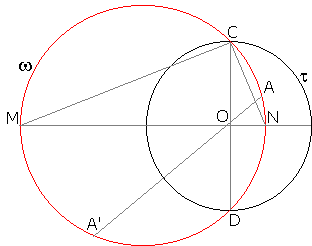## Inversion with Negative Power: What is this about? A Mathematical Droodle

### This applet requires Sun's Java VM 2 which your browser may perceive as a popup. Which it is not. If you want to see the applet work, visit Sun's website at https://www.java.com/en/download/index.jsp, download and install Java VM and enjoy the applet.

 What if applet does not run?

Explanation## Inversion with Negative Power

### This applet requires Sun's Java VM 2 which your browser may perceive as a popup. Which it is not. If you want to see the applet work, visit Sun's website at https://www.java.com/en/download/index.jsp, download and install Java VM and enjoy the applet.

 What if applet does not run?

The applet is supposed to remind of the symmetry in circle - inversion. The inverse image A' of a point A has the property that all circles perpendicular to the circle of inversion t and passing through A invariably pass through A'.

The current situation is slightly different:

 All circles through two diametrically opposite points on a given circle t and point A not at the center of t also pass through another point A'.

(In the applet the circle t is defined by its draggable center O and point R.)

### ProofLet CD be a diameter of t, w the circle through C, D and A, and let MN is the diameter of w that passes through O. CD is then perpendicular to MN.

ΔMCN is right, therefore

 (1) MO×NO = CO2 = OR2.

Let A' be the second point of intersection of OA with w. By the Intersecting Chords Theorem,

 (2) MO×NO = OA×OA'.

Combining (1) and (2) gives

 (3) OA×OA' = OR2,

which appears exactly like the inversion identity, but is not quite the same. The difference is in that formerly the points A and A' lay on the same side from O, while now O separates the two. If we consider OA and OA' as signed segments, then (3) should be corrected to

 (4) OA×OA' = -OR2.

The latter defines inversion with a negative power. The applet demonstrates the geometric meaning of such a transformation. Inversion in t with the power p < 0 is equivalent to the inversion with the power |p| and subsequent reflection in O.### Inversion - Introduction